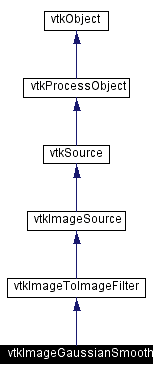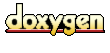Main Page   Class Hierarchy   Alphabetical List   Compound List   File List   Compound Members   File Members   Related Pages

# vtkImageGaussianSmooth Class Reference

Performs a gaussian convolution. More...

`#include <vtkImageGaussianSmooth.h>`

Inheritance diagram for vtkImageGaussianSmooth:[legend]
Collaboration diagram for vtkImageGaussianSmooth:[legend]
List of all members.

## Public Methods

virtual const char * GetClassName ()
virtual int IsA (const char *type)
void PrintSelf (ostream &os, vtkIndent indent)
virtual void SetStandardDeviations (float, float, float)
virtual void SetStandardDeviations (float)
void SetStandardDeviation (float std)
void SetStandardDeviations (float a, float b)
virtual float * GetStandardDeviations ()
virtual void GetStandardDeviations (float &, float &, float &)
virtual void GetStandardDeviations (float)
void SetStandardDeviation (float a, float b)
void SetStandardDeviation (float a, float b, float c)
virtual void SetRadiusFactors (float, float, float)
void SetRadiusFactors (float f, float f2)
virtual void GetRadiusFactors (float &, float &, float &)
virtual void SetDimensionality (int)
virtual int GetDimensionality ()

## Static Public Methods

int IsTypeOf (const char *type)
vtkImageGaussianSmooth * SafeDownCast (vtkObject *o)
vtkImageGaussianSmooth * New ()

## Protected Methods

vtkImageGaussianSmooth ()
~vtkImageGaussianSmooth ()
void ComputeKernel (double *kernel, int min, int max, double std)
void ComputeInputUpdateExtent (int inExt, int outExt)
void ExecuteAxis (int axis, vtkImageData *inData, int inExt, vtkImageData *outData, int outExt, int *pcycle, int target, int *pcount, int total)
void ThreadedExecute (vtkImageData *inData, vtkImageData *outData, int outExt, int id)

## Protected Attributes

int Dimensionality
float StandardDeviations 

## Detailed Description

Performs a gaussian convolution.

Date:
2001/11/13 14:32:35
Revision:
1.35
Thanks:
Thanks to C. Charles Law who developed this class.
vtkImageGaussianSmooth implements a convolution of the input image with a gaussian. Supports from one to three dimensional convolutions.
Tests:
vtkImageGaussianSmooth (Tests)

Definition at line 60 of file vtkImageGaussianSmooth.h.

## Constructor & Destructor Documentation

 vtkImageGaussianSmooth::vtkImageGaussianSmooth ( ) ` [protected]`

 vtkImageGaussianSmooth::~vtkImageGaussianSmooth ( ) ` [protected]`

## Member Function Documentation

 virtual const char* vtkImageGaussianSmooth::GetClassName ( ) ` [virtual]`
 Return the class name as a string. This method is defined in all subclasses of vtkObject with the vtkTypeMacro found in vtkSetGet.h. Reimplemented from vtkImageToImageFilter.

 int vtkImageGaussianSmooth::IsTypeOf ( const char * type ) ` [static]`
 Return 1 if this class type is the same type of (or a subclass of) the named class. Returns 0 otherwise. This method works in combination with vtkTypeMacro found in vtkSetGet.h. Reimplemented from vtkImageToImageFilter.

 virtual int vtkImageGaussianSmooth::IsA ( const char * type ) ` [virtual]`
 Return 1 if this class is the same type of (or a subclass of) the named class. Returns 0 otherwise. This method works in combination with vtkTypeMacro found in vtkSetGet.h. Reimplemented from vtkImageToImageFilter.

 vtkImageGaussianSmooth* vtkImageGaussianSmooth::SafeDownCast ( vtkObject * o ) ` [static]`
 Will cast the supplied object to vtkObject* is this is a safe operation (i.e., a safe downcast); otherwise NULL is returned. This method is defined in all subclasses of vtkObject with the vtkTypeMacro found in vtkSetGet.h. Reimplemented from vtkImageToImageFilter.

 void vtkImageGaussianSmooth::PrintSelf ( ostream & os, vtkIndent indent ) ` [virtual]`
 Methods invoked by print to print information about the object including superclasses. Typically not called by the user (use Print() instead) but used in the hierarchical print process to combine the output of several classes. Reimplemented from vtkImageToImageFilter.

 vtkImageGaussianSmooth* vtkImageGaussianSmooth::New ( ) ` [static]`
 Creates an instance of vtkImageGaussianSmmoth with the following defaults: Dimensioonality 3, StandardDeviations( 2, 2, 2), Radius Factors ( 1.5, 1.5, 1.5) Reimplemented from vtkImageToImageFilter.

 virtual void vtkImageGaussianSmooth::SetStandardDeviations ( float , float , float ) ` [virtual]`
 Sets/Gets the Standard deviation of the gaussian in pixel units.

 virtual void vtkImageGaussianSmooth::SetStandardDeviations ( float  ) ` [virtual]`
 Sets/Gets the Standard deviation of the gaussian in pixel units.

 void vtkImageGaussianSmooth::SetStandardDeviation ( float std ) ` [inline]`
 Sets/Gets the Standard deviation of the gaussian in pixel units. Definition at line 75 of file vtkImageGaussianSmooth.h.

 void vtkImageGaussianSmooth::SetStandardDeviations ( float a, float b ) ` [inline]`
 Sets/Gets the Standard deviation of the gaussian in pixel units. Definition at line 77 of file vtkImageGaussianSmooth.h.

 virtual float* vtkImageGaussianSmooth::GetStandardDeviations ( ) ` [virtual]`
 Sets/Gets the Standard deviation of the gaussian in pixel units.

 virtual void vtkImageGaussianSmooth::GetStandardDeviations ( float & , float & , float & ) ` [virtual]`
 Sets/Gets the Standard deviation of the gaussian in pixel units.

 virtual void vtkImageGaussianSmooth::GetStandardDeviations ( float  ) ` [virtual]`
 Sets/Gets the Standard deviation of the gaussian in pixel units.

 void vtkImageGaussianSmooth::SetStandardDeviation ( float a, float b ) ` [inline]`
 Sets/Gets the Standard deviation of the gaussian in pixel units. These methods are provided for compatibility with old scripts Definition at line 85 of file vtkImageGaussianSmooth.h.

 void vtkImageGaussianSmooth::SetStandardDeviation ( float a, float b, float c ) ` [inline]`
 Sets/Gets the Standard deviation of the gaussian in pixel units. These methods are provided for compatibility with old scripts Definition at line 87 of file vtkImageGaussianSmooth.h.

 virtual void vtkImageGaussianSmooth::SetRadiusFactors ( float , float , float ) ` [virtual]`
 Sets/Gets the Radius Factors of the gaussian in pixel units. The radius factors determine how far out the gaussian kernel will go before being clamped to zero.

 virtual void vtkImageGaussianSmooth::SetRadiusFactors ( float  ) ` [virtual]`
 Sets/Gets the Radius Factors of the gaussian in pixel units. The radius factors determine how far out the gaussian kernel will go before being clamped to zero.

 void vtkImageGaussianSmooth::SetRadiusFactors ( float f, float f2 ) ` [inline]`
 Sets/Gets the Radius Factors of the gaussian in pixel units. The radius factors determine how far out the gaussian kernel will go before being clamped to zero. Definition at line 96 of file vtkImageGaussianSmooth.h.

 void vtkImageGaussianSmooth::SetRadiusFactor ( float f ) ` [inline]`
 Sets/Gets the Radius Factors of the gaussian in pixel units. The radius factors determine how far out the gaussian kernel will go before being clamped to zero. Definition at line 97 of file vtkImageGaussianSmooth.h.

 virtual float* vtkImageGaussianSmooth::GetRadiusFactors ( ) ` [virtual]`
 Sets/Gets the Radius Factors of the gaussian in pixel units. The radius factors determine how far out the gaussian kernel will go before being clamped to zero.

 virtual void vtkImageGaussianSmooth::GetRadiusFactors ( float & , float & , float & ) ` [virtual]`
 Sets/Gets the Radius Factors of the gaussian in pixel units. The radius factors determine how far out the gaussian kernel will go before being clamped to zero.

 virtual void vtkImageGaussianSmooth::GetRadiusFactors ( float  ) ` [virtual]`
 Sets/Gets the Radius Factors of the gaussian in pixel units. The radius factors determine how far out the gaussian kernel will go before being clamped to zero.

 virtual void vtkImageGaussianSmooth::SetDimensionality ( int ) ` [virtual]`
 Set/Get the dimensionality of this filter. This determines whether a one, two, or three dimensional gaussian is performed.

 virtual int vtkImageGaussianSmooth::GetDimensionality ( ) ` [virtual]`
 Set/Get the dimensionality of this filter. This determines whether a one, two, or three dimensional gaussian is performed.

 void vtkImageGaussianSmooth::ComputeKernel ( double * kernel, int min, int max, double std ) ` [protected]`

 void vtkImageGaussianSmooth::ComputeInputUpdateExtent ( int inExt, int outExt ) ` [protected, virtual]`
 Reimplemented from vtkImageToImageFilter.

 void vtkImageGaussianSmooth::ExecuteAxis ( int axis, vtkImageData * inData, int inExt, vtkImageData * outData, int outExt, int * pcycle, int target, int * pcount, int total ) ` [protected]`

 void vtkImageGaussianSmooth::ThreadedExecute ( vtkImageData * inData, vtkImageData * outData, int outExt, int id ) ` [protected, virtual]`
 If the subclass does not define an Execute method, then the task will be broken up, multiple threads will be spawned, and each thread will call this method. It is public so that the thread functions can call this method. Reimplemented from vtkImageToImageFilter.

## Member Data Documentation

 int vtkImageGaussianSmooth::Dimensionality` [protected]`
 Definition at line 112 of file vtkImageGaussianSmooth.h.

 float vtkImageGaussianSmooth::StandardDeviations` [protected]`
 Definition at line 113 of file vtkImageGaussianSmooth.h.

 float vtkImageGaussianSmooth::RadiusFactors` [protected]`
 Definition at line 114 of file vtkImageGaussianSmooth.h.

The documentation for this class was generated from the following file:

Generated on Thu Mar 28 14:30:35 2002 for VTK by1.2.11.1 written by Dimitri van Heesch, © 1997-2001